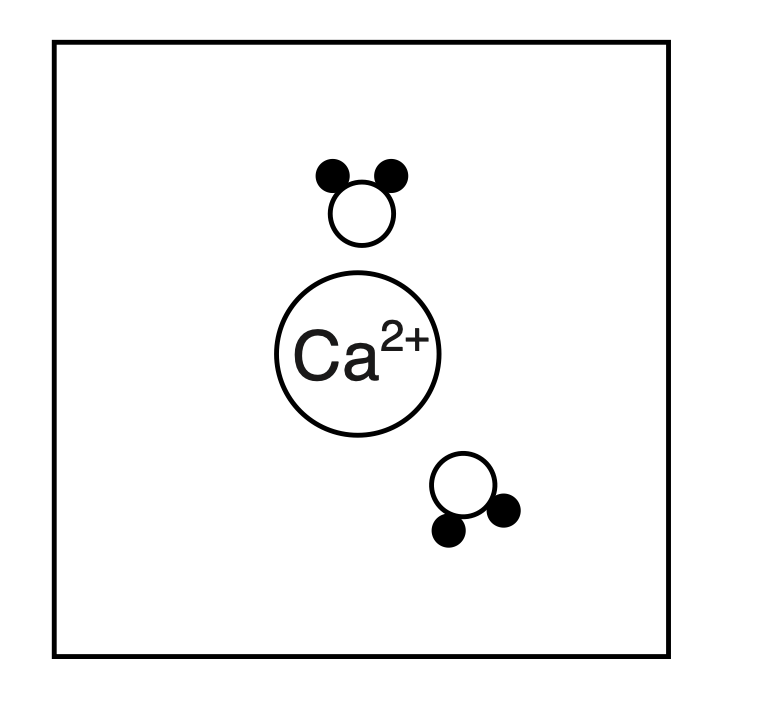top of page
Search

# One sample of tap water contains dissolved ions such as Ca2+(aq), Mg2+(aq), and CO32+(aq). A 150.-gr

NY Regents Chemistry Exam June 2022

One sample of tap water contains dissolved ions such as Ca^2+(aq), Mg^2+(aq), and CO3^2+(aq). A 150.-gram sample of this tap water contains 0.00075 gram of CaCO3(aq). When these ions in the tap water are present in greater concentrations, the water is called hard water. The hard water can damage water pipes and water heaters by producing large deposits of solid calcium carbonate, known as scale. Some homeowners have a water softener to replace positive ions, such as Ca^2+(aq) and Mg^2+(aq), in hard water with sodium ions, Na^+(aq).

71 Determine the parts per million of CaCO3 in the tap water sample. 

Explanation: Table T on the Reference Table has an equation for parts per million calculation which is

Parts per million = (mass of solute/ mass of solution) * 1 000 000

We can now plug in the numbers, Parts per million of CaCO3 = (0.00075 gram/ 150g)*1 000 000

72 State, in terms of aqueous ions, why this tap water can conduct an electric current. 

Explanation: In order to conduct electricity we need charged particles moving such as aqueous ions.

Answer: Tap water contains aqueous ions which allows it to conduct an electric current

73 Using the key in your answer booklet, draw at least two water molecules in the box, showing the orientation of each water molecule toward the Ca^2+ ion.Explanation: A water molecule consists of two hydrogens and one oxygen. Oxygen is partially negative and hydrogens are partially positive. Negative charge is attached to positive charge. Oxygen would be oriented towards the positively charge Ca^2+ ion.